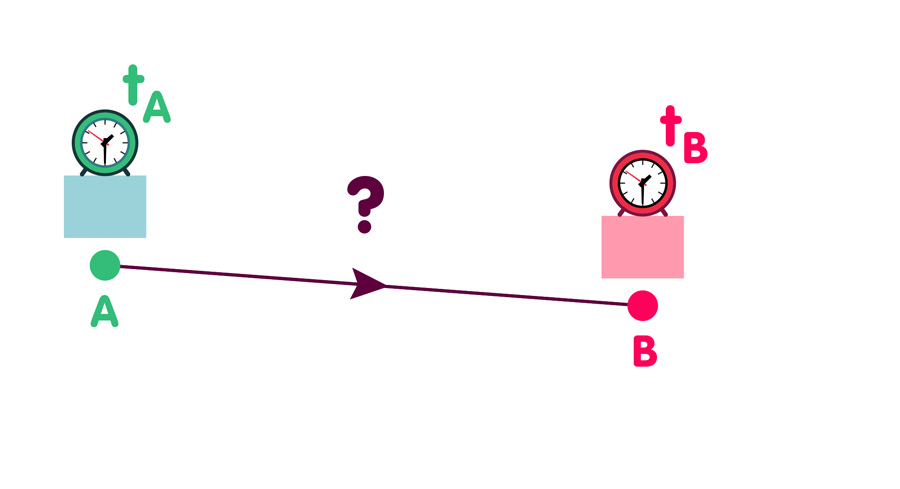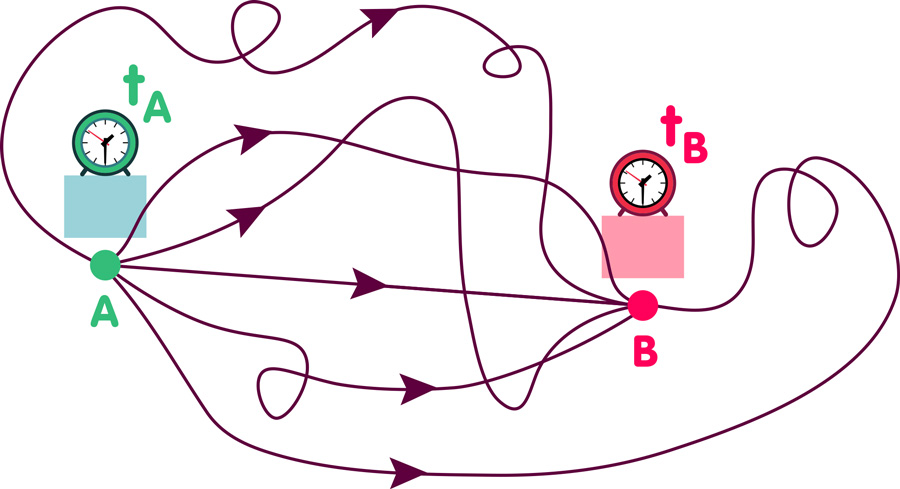# The sum over all possibilities: The path integral formulation of quantum theory

### About the path integral approach to quantum theory

#### An article by Markus Pössel

A fundamental difference between classical physics and quantum theory is the fact that, in the quantum world, certain predictions can only be made in terms of probabilities

## A travelling particle

As an example, take the question whether or not a particle that starts at the time tA at the location A will reach location B at the later time tB.Classical physics can give a definite answer. Depending on the particle’s initial velocity and the forces acting on it, the answer is either yes or no. In quantum theory, it is merely possible to give the probability that the particle in question can be detected at location B at time tB.

The path integral formalism, which was invented by the US physicist Richard Feynman, is a tool for calculating such quantum mechanical probabilities. Feynman’s recipe, applied to a particle travelling from A to B, is the following.

Step 1: Consider all possibilities for the particle travelling from A to B. Not only the boring straight-line approach, but also the possibility of the particle turning loopings and making diverse detours:The illustration hardly does the particle justice. It shows a mere six from an infinity of possibilities. It neglects to show the cases in which the particle visits New York, Ulan Bator, or even the moon or the Andromeda Galaxy before arriving at its destination. Last but not least, it does not contain information about velocities. The first part of the particle’s trajectory may be travelled at break-neck speed and the final millimetres at a snail’s pace – or the other way around, or completely different; another infinity of possibilities. In short, for the first step, take into account all ways of travelling from A to B, however outlandish they may seem.

The second step is to associate a number with each of these possibilities (not quite the kind of number we’re used to from school, but we will not bother with the difference here). Finally, the numbers associated with all possibilities are added up – some parts of the sum canceling each other, others adding up. (Readers whom this makes think of waves are on the right track – it is an example of an interference phenomenon.) The resulting sum tells us the probability of detecting the particle that started out at A at the location B at the specified time. Physicists call such a sum over all possibilities a path integral or sum over histories.

## A different kind of time

However, actually calculating such path integrals can be rather tricky. As an example, take particle physics, whose theories are a combination of quantum theory and special relativity. There, path integrals are an important tool to calculate the probability of particles interacting with each other in a given way. But there’s a catch, and in order to actually perform such calculations, one needs to resort to a mathematical trick: Everywhere that Feynman’s formula contains the time coordinate t, this t gets an extra factor i. Here, i is the so-called “imaginary unit”, an algebraic symbol that, by definition, squares to minus one,i2=-1. (The resulting pair i·t is sometimes called “imaginary time”.) After the path integral calculation is done, one reverses this substitution.

The replacement might seem artificial and implausible. In a way, it corresponds to transforming the time coordinate into just another space coordinate. Fact is, it makes the Feynman recipe give the right answers. There’s even an exact proof, found by two mathematical physicists, Konrad Osterwalder from Switzerland and the German Robert Schrader: They proved a theorem showing that the properties of a quantum theory formulated in the spacetime of special relativity can indeed be reconstructed exactly by using the Feynman recipe on an imaginary-time version of that same spacetime.

## Path integrals and quantum gravity

Path integrals also play a role in some of the candidate theories for a theory of quantum gravity. In string theory, for instance, the probability of given string interactions can be calculated as a path integral – in addition to all possibilities a string can travel through space, the sum is over all possibilities the string can deform and wiggle on the way.

Also, in the context of what is called quantum cosmology, some proposals for how the universe might have begun (including its “initial state”) are formulated using path integrals, with or without the mysterious factor i. In this framework, the probability of the universe evolving into a certain state results from the sum over all possibilities for how this evolution might take place – literally a sum over all possible histories of the universe from its initial state to the present. However, the mathematical basis for defining path integrals where the curved spacetime of general relativity comes into play leads to some problems that, as of yet, have not been resolved completely.

## Further Information

Some basics of relativistic particle physics and quantum gravity can be found in Elementary Einstein in the section Relativity and the quantum.

Quantum cosmology based on path integrals is described in the spotlight topic Searching for the quantum beginning of the universe. Related Spotlights on relativity can be found in the section Relativity and the quantum.

###### Colophon
Markus Pössel

is the managing scientist at Haus der Astronomie, the Center for Astronomy Education and Outreach in Heidelberg, and senior outreach scientist at the Max Planck Institute for Astronomy. He initiated Einstein Online.